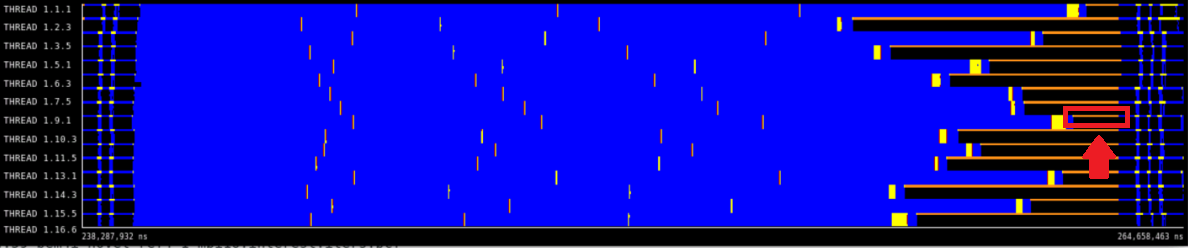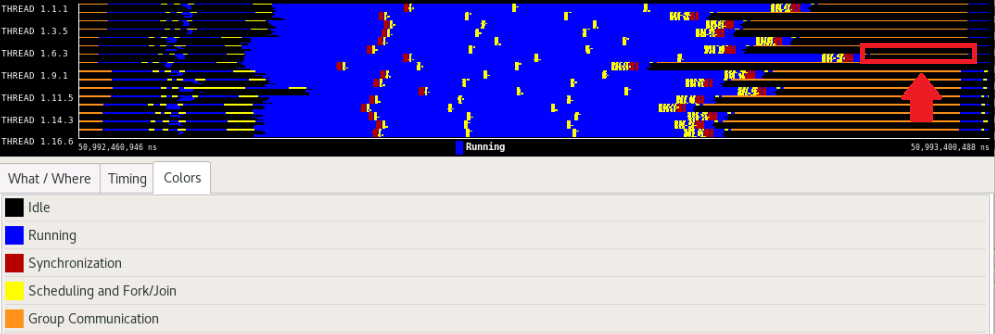# Low transfer efficiency following large computation

Usual symptom(s):
• Transfer Efficiency: The Transfer Efficiency (TE) measures inefficiencies due to time in data transfer. (more...)

Many parallel algorithms on distributed memory systems contain a certain pattern, where a computational phase is serially followed by collective communication to share computed results. Moreover, this is often present in an iterative process, thus this pattern repeats in the algorithm many times.

...
compute(); // long computation phase
communicate(); // communication phase
...


Based on the ratio between both phases we can distinguish cases where this pattern has nothing to worry about, and cases where we could apply some of the techniques to overlap both phases in time.

Time spent by a communication phase relies on several aspects. The most important is what amount of data is being transferred. The fewer data an application transfers, the better. Scalars, vectors, or matrices of constant and small size should be fine in most cases. When thinking of larger matrices or very large vectors, we might get the ratio between communication and computation phase higher. The overall number of processes within the communication plays also an important role, whether it is a point-to-point, all-to-all, or other collective communication. If the computational phase scales well up to thousands of processes, the time needed to perform for example a collective reduction operation with such many processes may start to dominate.

HW Co-design: If the network bandwidth increases, it will mitigate the effect of this pattern, because the time spent in the communication will decrease.

A potential problem in this pattern is serialized computation and communication. During the communication phase, we can identify stalled resources. The effect is more distinct when using multiple threads per process (OpenMP, pthreads). In POP metrics, the Communication Efficiency reflects the loss of efficiency by communication. It is based on the Transfer Efficiency which measures the ratio between the total runtime on an ideal network and the total measured runtime. When the communication phase is not insignificant, these two efficiencies will point it out.

Here we demonstrate the pattern on two measurements from the BEM4I application. The Running stage in the timeline corresponds to the computation phase compute(). In the first trace (Scenario 1), the communication phase (highlighted by the red arrow) takes roughly around 8% of the runtime and the Communication efficiency is above 95%. In the second trace (Scenario 2), the ratio between computation and communication changed. The highlighted part takes around 20% and the Communication efficiency is 76%.Related efficiencies are:

Scenario 1 Scenario 2
Parallel eff. 0.78 0.51
- Load Balance 0.82 0.67
- Communication eff. 0.95 0.76
- Serialization eff. 0.96 0.97
- Transfer eff. 0.99 0.78

This pattern can be also typical for offloaded computing where intensive computational parts of some codes are offloaded to devices/hardware accelerators such as Nvidia GPUs, Intel Xeon Phis, and others. For such applications, where data is often quite large, an optimization technique is of importance.

### Variants based on dependencies

We can distinguish different use cases. When the communication and computation does not raise any kind of data dependence among them, that is:

compute(data_A);
...
compute(data_B);
communicate(data_A);


We must also consider the case in which the communication phase involves the data it has been computed immediately before:

...
compute(data_A);
communicate(data_A);

Recommended best-practice(s):